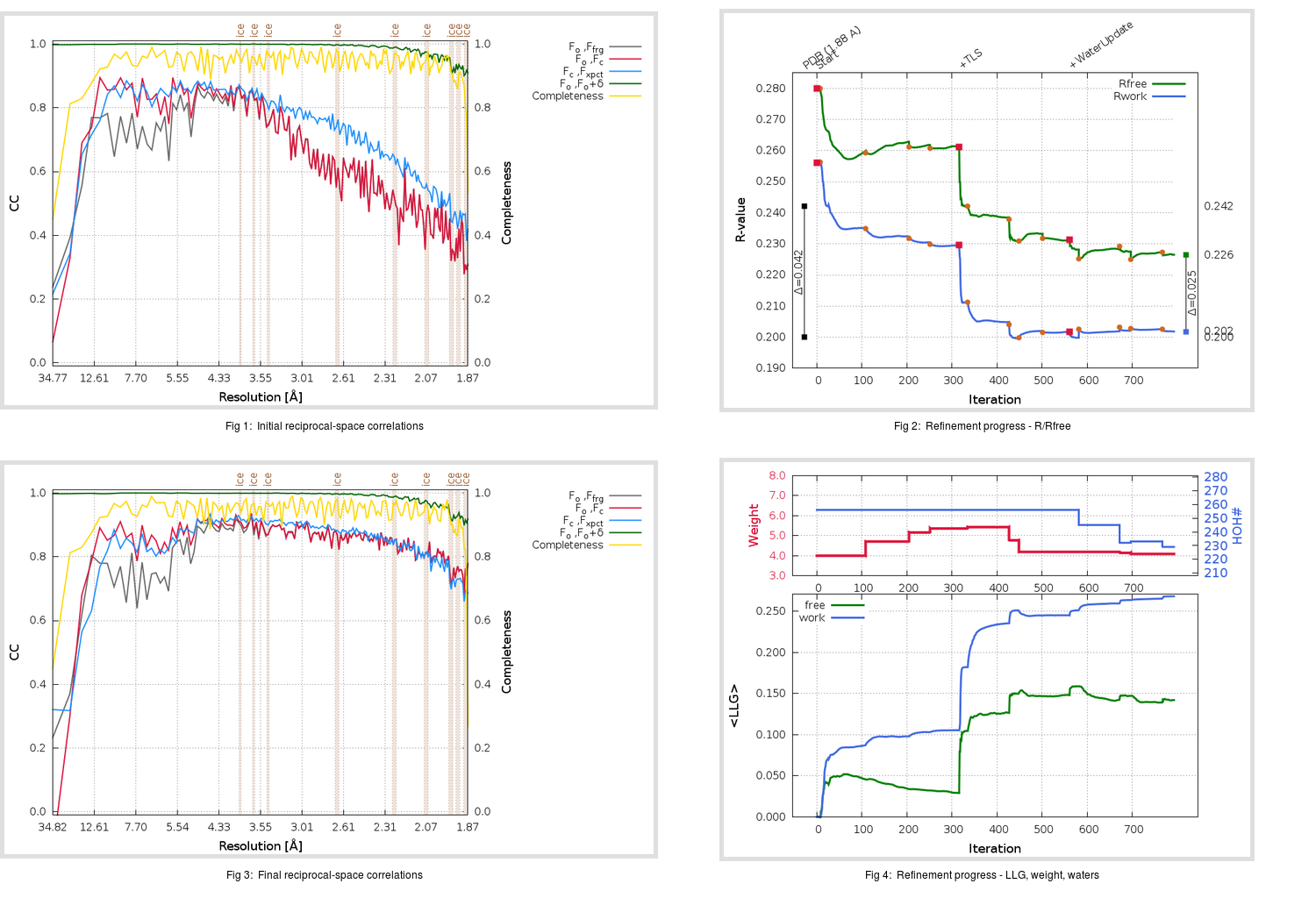Content:

```    Diffraction limits & principal axes of ellipsoid fitted to diffraction cut-off surface:
1.844         0.7660   0.0000   0.6428       0.966 a* + 0.258 c*
1.858         0.0000   1.0000   0.0000       b*
1.807        -0.6428   0.0000   0.7660      -0.818 a* + 0.575 c*
```

## Deposited

` `
 Date deposited Date data collection Resolution R, Rfree 20050630 20041117 1.88 0.1980 0.2420

Molprobity (CCP4 7.0 version) summary:

```Ramachandran outliers =   0.66 %
favored =  96.38 %
Rotamer outliers      =   9.71 %
C-beta deviations     =    10
Clashscore            =  23.29
RMS(bonds)            =   0.0224
RMS(angles)           =   2.35
MolProbity score      =   2.85
Resolution            =   1.88
R-work                =   0.1980
R-free                =   0.2420
```

```Number of waters      =   256

<B> (all atoms) =   44.00 ( sd =    8.47 ) for       2752 non-hydrogen atoms
<B>   (protein) =   42.72 ( sd =    7.12 ) for       2436 non-hydrogen atoms
<B>     (water) =   55.91 ( sd =    9.34 ) for        256 non-hydrogen atoms
<B>    (others) =   43.86 ( sd =   11.58 ) for         60 non-hydrogen atoms

B min/max       (all non-hydrogen atoms) =   24.99 /   76.17
B min/max   (protein non-hydrogen atoms) =   24.99 /   72.31
B min/max     (water non-hydrogen atoms) =   27.63 /   76.17
B min/max     (other non-hydrogen atoms) =   29.27 /   55.03
```

## BUSTER (re-)refinement

` `

Molprobity (CCP4 7.0 version) summary:

```Ramachandran outliers =   0.66 %
favored =  96.71 %
Rotamer outliers      =   3.96 %
C-beta deviations     =     1
Clashscore            =   5.02
RMS(bonds)            =   0.0114
RMS(angles)           =   1.62
MolProbity score      =   1.93
Resolution            =   1.87
R-work                =   0.2018
R-free                =   0.2265
```

```Number of waters      =   229

<B> (all atoms) =   50.49 ( sd =   14.06 ) for       2725 non-hydrogen atoms
<B>   (protein) =   49.36 ( sd =   13.39 ) for       2436 non-hydrogen atoms
<B>     (water) =   63.65 ( sd =   13.81 ) for        229 non-hydrogen atoms
<B>    (others) =   44.49 ( sd =   11.56 ) for         60 non-hydrogen atoms

B min/max       (all non-hydrogen atoms) =   27.50 /  103.12
B min/max   (protein non-hydrogen atoms) =   27.50 /   89.12
B min/max     (water non-hydrogen atoms) =   27.97 /  103.12
B min/max     (other non-hydrogen atoms) =   29.00 /   55.04
```

Refinement progression:Results:

` `
 File Remark 2A5I_aB_refine.01_03_refine.pdb.gz exact refinement commands are in header 2A5I_aB_refine.01_03_refine.mtz.gz including original deposited data and several re-refinement map coefficients 2A5I_aB_refine.01_03_BUSTER_model.cif.gz including any non-standard compound restraints 2A5I_aB_refine.01_03_BUSTER_refln.cif.gz